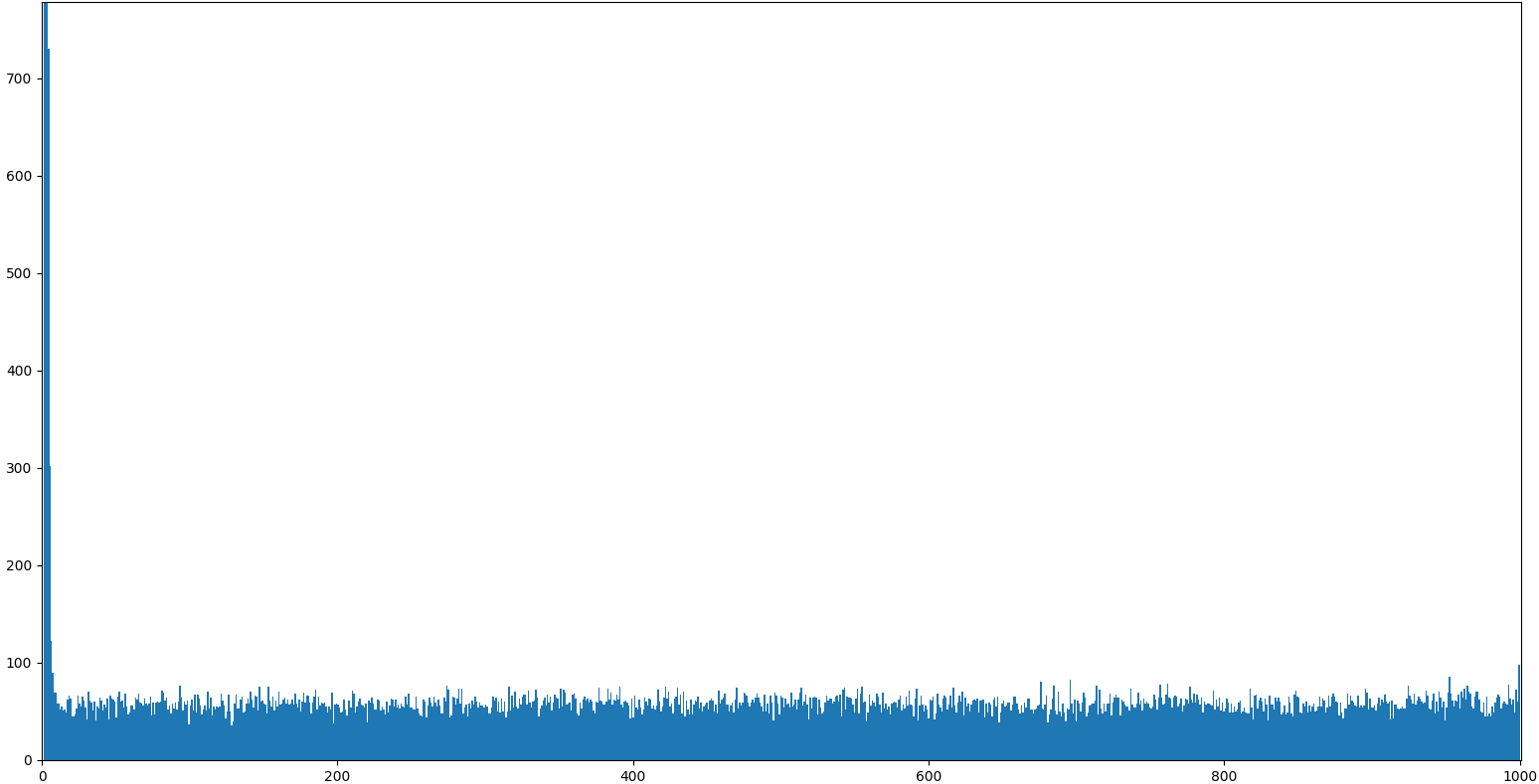# samplitude Release 0.2.0

Samplitude (s8e) is a statistical distributions command line tool

Keywords
jinja2, jinja, random, statistics, sample, distribution, plot
GPL-3.0/GPL-3.0+
Install
``` pip install samplitude==0.2.0 ```

# SamplitudeCLI generation and plotting of random variables:

```\$ samplitude "sin(0.31415) | sample(6) | round | cli"
0.0
0.309
0.588
0.809
0.951
1.0```

The word samplitude is a portmanteau of sample and amplitude. This project also started as an étude, hence should be pronounced sampl-étude.

`samplitude` is a chain starting with a generator, followed by zero or more filters, followed by a consumer. Most generators are infinite (with the exception of `range` and `lists` and possibly `stdin`). Some of the filters can turn infinite generators into finite generators (like `sample` and `gobble`), and some filters can turn finite generators into infinite generators, such as `choice`.

Consumers are filters that necessarily flush the input; `list`, `cli`, `json`, `unique`, and the plotting tools, `hist`, `scatter` and `line` are examples of consumers. The `list` consumer is a Jinja2 built-in, and other Jinja2 consumers are `sum`, `min`, and `max`:

```samplitude "sin(0.31415) | sample(5) | round | max | cli"
0.951```

For simplicity, s8e is an alias for samplitude.

## Generators

In addition to the standard `range` function, we support infinite generators

• `exponential(lambd)`: `lambd` is 1.0 divided by the desired mean.
• `uniform(a, b)`: Get a random number in the range `[a, b)` or `[a, b]` depending on rounding.
• `gauss(mu, sigma)`: `mu` is the mean, and `sigma` is the standard deviation.
• `normal(mu, sigma)`: as above
• `lognormal(mu, sigma)`: as above
• `triangular(low, high)`: Continuous distribution bounded by given lower and upper limits, and having a given mode value in-between.
• `beta(alpha, beta)`: Conditions on the parameters are `alpha > 0` and `beta > 0`. Returned values range between 0 and 1.
• `gamma(alpha, beta)`: as above
• `weibull(alpha, beta)`: `alpha` is the scale parameter and `beta` is the shape parameter.
• `pareto(alpha)`: Pareto distribution. `alpha` is the shape parameter.
• `vonmises(mu, kappa)`: `mu` is the mean angle, expressed in radians between 0 and `2*pi`, and `kappa` is the concentration parameter, which must be greater than or equal to zero. If kappa is equal to zero, this distribution reduces to a uniform random angle over the range 0 to `2*pi`.

Provided that you have installed the `scipy.stats` package, the

• `pert(low, peak, high)` distribution is supported.

We have a special infinite generator (filter) that works on finite generators:

• `choice`,

whose behaviour is explained below.

For input from files, either use `words` with a specified environment variable `DICTIONARY`, or pipe through

• `stdin()`

which reads from `stdin`.

If the file is a csv file, there is a `csv` generator that reads a csv file with Pandas and outputs the first column (if nothing else is specified). Specify the column with either an integer index or a column name:

```>>> samplitude "csv('iris.csv', 'virginica') | counter | cli"
0 50
1 50
2 50```

For other files, we have the `file` generator:

```>>> s8e "file('iris.csv') | sample(1) | cli"
150,4,setosa,versicolor,virginica```

Finally, we have `combinations` and `permutations` that are inherited from itertools and behave exactly like those.

`>>> s8e "'ABC' | permutations | cli"`

However, the output of this is rather non-UNIXy, with the abstractions leaking through:

```>>> s8e "'HT' | permutations | cli"
('H', 'T')
('T', 'H')```

So to get a better output, we can use an elementwise join `elt_join`:

```>>> s8e "'HT' | permutations | elt_join | cli"
H T
T H```

which also takes a seperator as argument:

```>>> s8e "'HT' | permutations | elt_join(';') | cli"
H;T
T;H```

This is already supported by Jinja's `map` function (notice the strings around `join`):

```>>> s8e "'HT' | permutations | map('join', ';') | cli"
H;T
T;H```

We can thus count the number of permutations of a set of size 10:

```>>> s8e "range(10) | permutations | len"
3628800```

The `product` generator takes two generators and computes a cross-product of these. In addition,

## A warning about infinity

All generators are (potentially) infinite generators, and must be sampled with `sample(n)` before consuming!

## Usage and installation

Install with

`pip install samplitude`

or to get bleeding release,

`pip install git+https://github.com/pgdr/samplitude`

### Examples

This is pure Jinja2:

```>>> samplitude "range(5) | list"
[0, 1, 2, 3, 4]```

However, to get a more UNIXy output, we use `cli` instead of `list`:

```>>> s8e "range(5) | cli"
0
1
2
3
4```

To limit the output, we use `sample(n)`:

```>>> s8e "range(1000) | sample(5) | cli"
0
1
2
3
4```

That isn't very helpful on the `range` generator, which is already finite, but is much more helpful on an infinite generator. The above example is probably better written as

```>>> s8e "count() | sample(5) | cli"
0
1
2
3
4```

However, much more interesting are the infinite random generators, such as the `uniform` generator:

```>>> s8e "uniform(0, 5) | sample(5) | cli"
3.3900198868059235
1.2002767137709318
0.40999391897569126
1.9394585953696264
4.37327472704115```

We can round the output in case we don't need as many digits (note that `round` is a generator as well and can be placed on either side of `sample`):

```>>> s8e "uniform(0, 5) | round(2) | sample(5) | cli"
4.98
4.42
2.05
2.29
3.34```

### Selection and modifications

The `sample` behavior is equivalent to the `head` program, or from languages such as Haskell. The `head` alias is supported:

```>>> samplitude "uniform(0, 5) | round(2) | head(5) | cli"
4.58
4.33
1.87
2.09
4.8```

`drop` is also available:

```>>> s8e "uniform(0, 5) | round(2) | drop(2) | head(3) | cli"
1.87
2.09
4.8```

To shift and scale distributions, we can use the `shift(s)` and `scale(s)` filters. To get a Poisson distribution process starting at 15, we can run

```>>> s8e "poisson(4) | shift(15) | sample(5) |cli"
18
21
19
22
17```

or to get the Poisson point process (exponential distribution),

```>>> s8e "exponential(4) | round | shift(15) | sample(5) |cli"
16.405
15.54
15.132
15.153
15.275```

Both `shift` and `scale` work on generators, so to add `sin(0.1)` and `sin(0.2)`, we can run

`>>> s8e "sin(0.1) | shift(sin(0.2)) | sample(10) | cli"`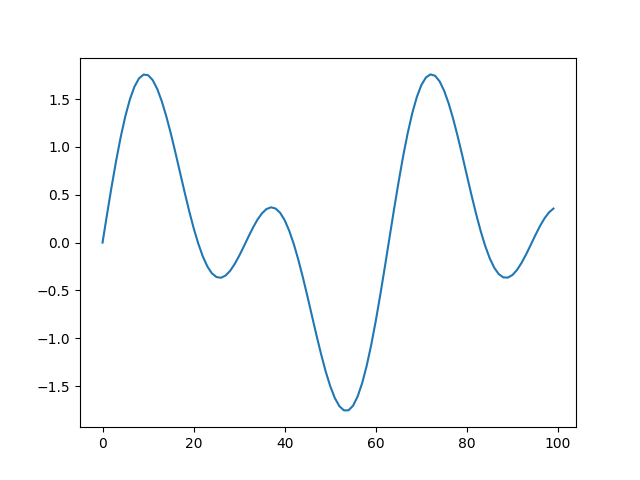### Choices and other operations

Using `choice` with a finite generator gives an infinite generator that chooses from the provided generator:

```>>> samplitude "range(0, 11, 2) | choice | sample(6) | cli"
8
0
8
10
4
6```

Jinja2 supports more generic lists, e.g., lists of strings. Hence, we can write

```>>> s8e "['win', 'draw', 'loss'] | choice | sample(6) | sort | cli"
draw
draw
loss
loss
loss
win```

... and as in Python, strings are also iterable:

```>>> s8e "'HT' | cli"
H
T```

... so we can flip six coins with

```>>> s8e "'HT' | choice | sample(6) | cli"
H
T
T
H
H
H```

We can flip 100 coins and count the output with `counter` (which is `collections.Counter`)

```>>> s8e "'HT' | choice | sample(100) | counter | cli"
H 47
T 53```

The `sort` functionality works as expected on a `Counter` object (a `dict` type), so if we want the output sorted by key, we can run

```>>> s8e "range(1,7) | choice | sample(100) | counter | sort | elt_join | cli" 42 # seed=42
1 17
2 21
3 12
4 21
5 13
6 16```

There is a minor hack to sort by value, namely by `swap`-ing the Counter twice:

```>>> s8e "range(1,7) | choice | sample(100) |
counter | swap | sort | swap | elt_join | cli" 42 # seed=42
3 12
5 13
6 16
1 17
2 21
4 21```

The `swap` filter does an element-wise reverse, with element-wise reverse defined on a dictionary as a list of `(value, key)` for each key-value pair in the dictionary.

So, to get the three most common anagram strings, we can run

```>>> s8e "words() | map('sort') | counter | swap | sort(reverse=True) |
swap | sample(3) | map('first') | elt_join('') | cli"
aeprs
acerst
opst```

Using `stdin()` as a generator, we can pipe into `samplitude`. Beware that `stdin()` flushes the input, hence `stdin` (currently) does not work with infinite input streams.

```>>> ls | samplitude "stdin() | choice | sample(1) | cli"
some_file```

Then, if we ever wanted to shuffle `ls` we can run

```>>> ls | samplitude "stdin() | shuffle | cli"
some_file```
```>>> cat FILE | samplitude "stdin() | cli"
# NOOP; cats FILE```

### The fun powder plot

For fun, if you have installed `matplotlib`, we support plotting, `hist` being the most useful.

`>>> samplitude "normal(100, 5) | sample(1000) | hist"`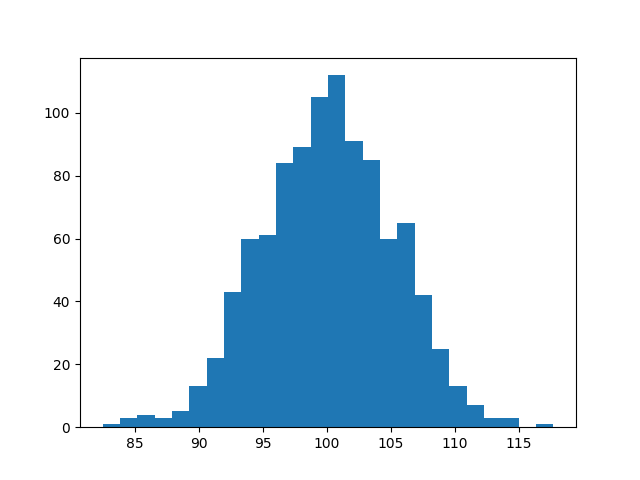An exponential distribution can be plotted with `exponential(lamba)`. Note that the `cli` output must be the last filter in the chain, as that is a command-line utility only:

`>>> s8e "normal(100, 5) | sample(1000) | hist | cli"`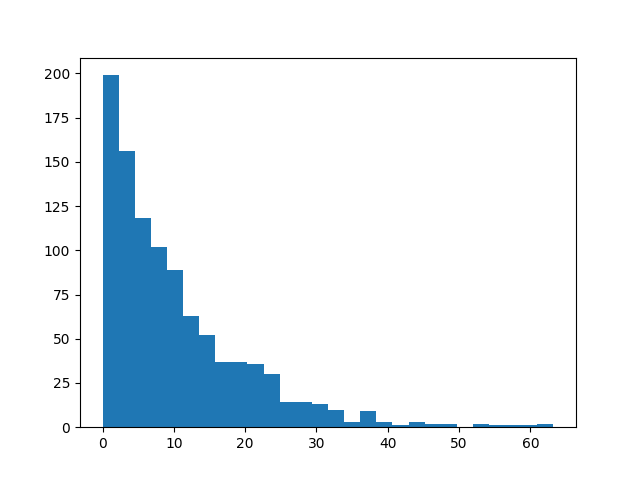To repress output after plotting, you can use the `gobble` filter to empty the pipe:

`>>> s8e "normal(100, 5) | sample(1000) | hist | gobble"`

The `pert` distribution takes inputs `low`, `peak`, and `high`:

`>>> s8e "pert(10, 50, 90) | sample(100000) | hist(100) | gobble"`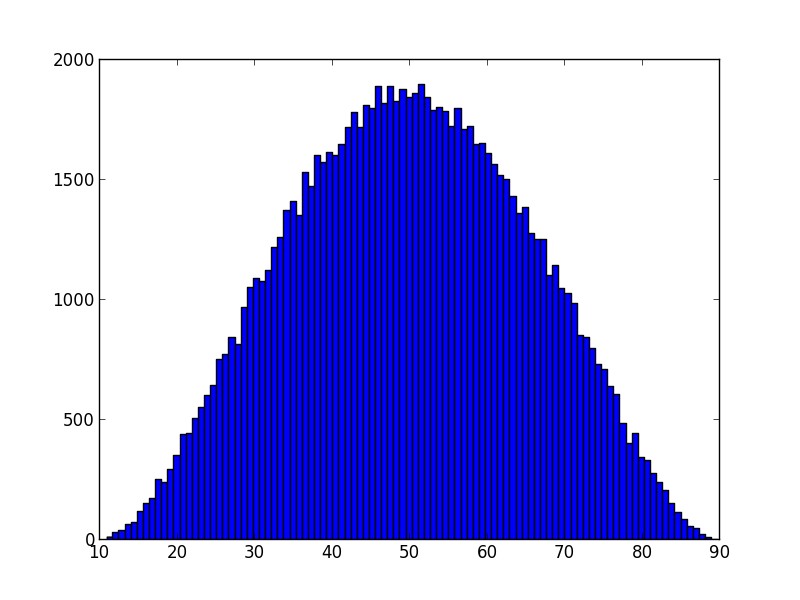Although `hist` is the most useful, one could imaging running `s8e` on timeseries, where a `line` plot makes most sense:

`>>> s8e "sin(22/700) | sample(200) | line"`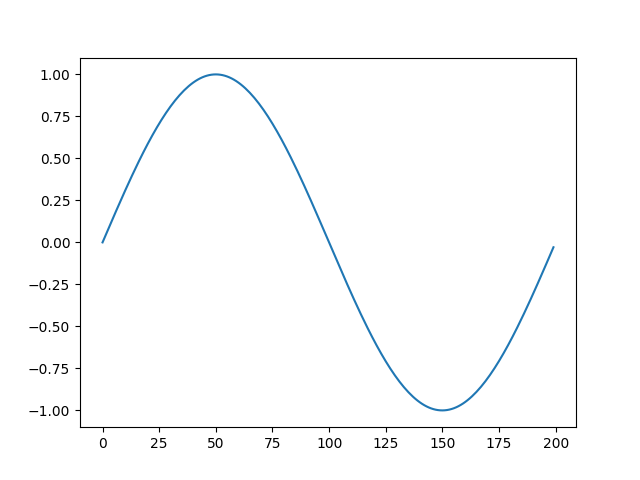The scatter function can also be used, but requires that the input stream is a stream of pairs, which can be obtained either by the `product` generator, or via the `pair` or `counter` filter:

`s8e "normal(100, 10) | sample(10**5) | round(0) | counter | scatter"`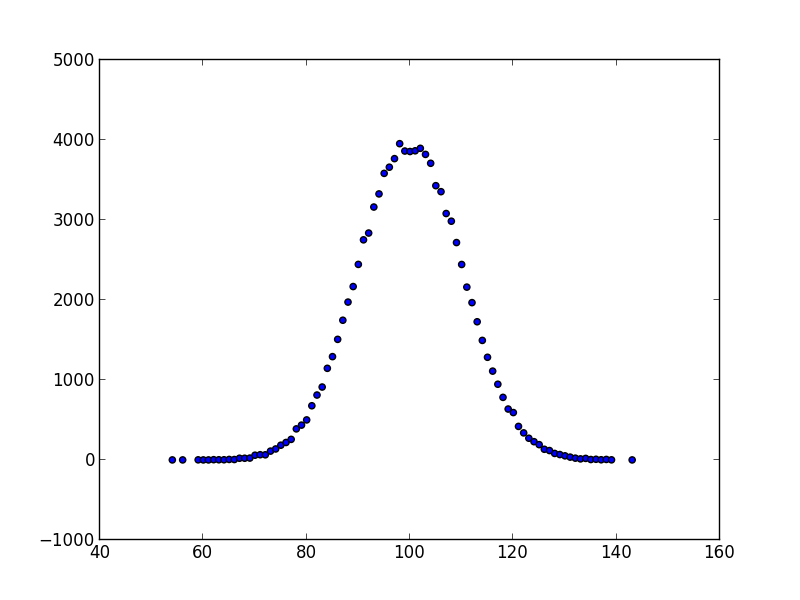### Fourier

A fourier transform is offered as a filter `fft`:

`>>> samplitude "sin(0.1) | shift(sin(0.2)) | sample(1000) | fft | line | gobble"`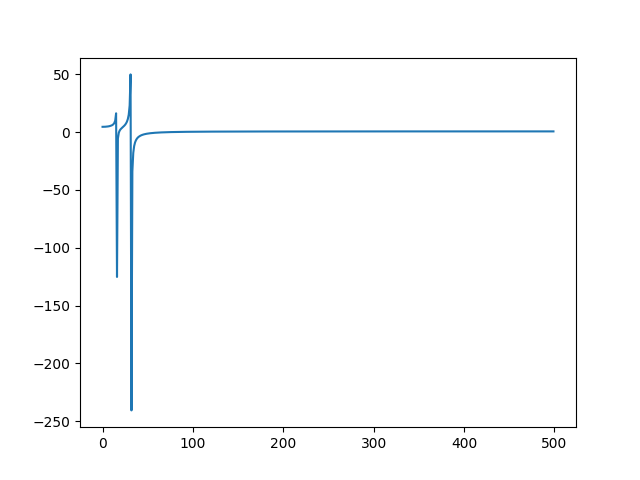## Your own filter

If you use Samplitude programmatically, you can register your own filter by sending a dictionary

```{'name1' : filter1,
'name2' : filter2,
#...,
'namen' : filtern,
}```

to the `samplitude` function.

### Example: secretary problem

Suppose you want to emulate the secretary problem ...

#### Intermezzo: The problem

For those not familiar, you are a boss, Alice, who wants to hire a new secretary Bob. Suppose you want to hire the tallest Bob of all your candidates, but the candidates arrive in a stream, and you know only the number of candidates. For each candidate, you have to accept (hire) or reject the candidate. Once you have rejected a candidate, you cannot undo the decision.

The solution to this problem is to look at the first `n/e` (`e~2.71828` being the Euler constant) candidates, and thereafter accept the first candidate taller than all of the `n/e` first candidates.

#### A Samplitude solution

Let `normal(170, 10)` be the candidate generator, and let `n=100`. We create a filter `secretary` that takes a stream and an integer (`n`) and picks according to the solution. In order to be able to assess the quality of the solution later, the filter must forward the entire list of candidates; hence we annotate the one we choose with `(c, False)` for a candidate we rejected, and `(c, True)` denotes the candidate we accepted.

```def secretary(gen, n):
import math
explore = int(n / math.e)
target = -float('inf')
i = 0

# explore the first n/e candidates
for c in gen:
target = max(c, target)
yield (c, False)
i += 1
if i == explore:
break

_ok = lambda c, i, found: ((i == n-1 and not found)
or (c > target and not found))

have_hired = False
for c in gen:
status = _ok(c, i, have_hired)
have_hired = have_hired or status
yield c, status
i += 1
if i == n:
return```

Now, to emulate the secretary problem with Samplitude:

```from samplitude import samplitude as s8e

# insert above secretary function

n = 100
filters = {'secretary': secretary}

solution = s8e('normal(170, 10) | secretary(%d) | list' % n, filters=filters)
solution = eval(solution)  # Samplitude returns an eval-able string
cands = map(lambda x: x, solution)
opt = [s for s in solution if s]
# the next line prints in which position the candidate is
print(1+sorted(cands, reverse=True).index(opt), '/', n)```

In about 67% of the cases we can expect to get one of the top candidates, whereas the remaining 33% of the cases will be uniformly distributed. Running 100k runs with a population of size 1000 reveals the structure.